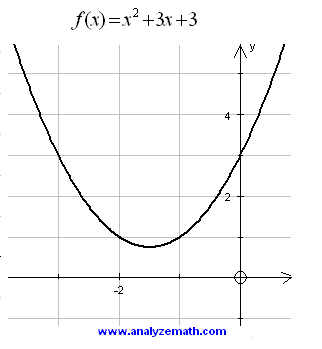# Graphs of Second Degree Polynomials

The graphs of several second degree polynomials are shown along with questions and answers at the bottom of the page.

1. Polynomial of a second degree polynomial: touches the x axis and upward.
Question 1: Why does the parabola open upward? Why does it touch the x axis at one point only?Figure 1: Graph of a second degree polynomial.

2. Polynomial of a second degree polynomial: two x intercepts and upward.
Question 2: Why does the parabola cut the x axis at two distinct points?Figure 2: Graph of a second degree polynomial

3. Polynomial of a second degree polynomial: two x intercepts and downward
Question 3: Why does the parabola open downward?Figure 3: Graph of a second degree polynomial

4. Polynomial of a second degree polynomial: no x intercept and upward
Question 4: Why does the graph have no x-intercepts?Figure 4: Graph of a second degree polynomial